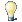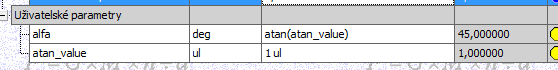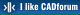#CAD tip # 9248:%  platform  category## Math functions in parameters of Inventor models.You can use many arithmetic and goniometric functions in calculated paramaters of Inventor models:Supported algebraic operators:
```
- subtraction
% modulo
* multiplication
/ division
^ power
() expression delimiter
; argument delimiter
```

Supported function:

```
cos(expr)
sin(expr)
tan(expr)
acos(expr)
asin(expr)
atan(expr)
cosh(expr)
sinh(expr)
tanh(expr)
acosh(expr)
asinh(expr)
atanh(expr)
sqrt(expr) - square root
sign(expr) - signum 0/1
exp(expr) - e^x
floor(expr) - next lower integer
ceil(expr) - next higher integer
round(expr) - round to integer
abs(expr)
max(expr1;expr2)
min(expr1;expr2)
ln(expr) - natural logarithm
log(expr) - decimal logarithm
pow(expr1; expr2)
random() - random number
isolate(expr;unit;unit)
```

Please note that all expressions must be unit-compatible.

 Inventor2014*  CAD 14.5.2013    18309×applies to: Inventor 2014 ·

 See also: Tip 12777: Arithmetic and trigonometric functions available in Revit expressions. Tip 12749: Generate parametric mathematical 3D surfaces (meshes) in Fusion 360. Tip 12638: How to use conditional parameter values in Fusion 360? Tip 12600: Parametric 3D curves in Fusion 360. Tip 11727: Animated drawing of a curve shape in AutoCAD.

Back   All CAD TipsHave we helped you? If you want to support the CAD Forum web service, consider buying one of our CAD applications, or our custom software development offerings, or donating via PayPal (see above). You may also add a link to your web - like this "fan" link:Featuring:Track your time spent working on AutoCAD or Revit jobs - automatically with CADstudio JobTime